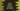# Java program to check for disjoint sets in two ways## How to check if two sets are disjoint in Java:

In this post, we will learn how to check if two sets are disjoint or not in Java. Two sets are called disjoint sets if the sets have no common elements. The intersection of two disjoint sets is an empty set. For example, {1, 2, 3} and {4, 5} are disjoint sets. But, {1, 2, 3} and {3, 4} are not disjoint sets.

### Example 1: Iterative Java program to check if two sets are disjoint:

In this method, we will iterate over the elements of one set and for each element we will check if the second set contains it or not. If it does, it will return false i.e. these are not disjoint sets. Else, it will return true.

Let’s take a look at the example:

``````import java.util.*;

public class Main {
public static <E> boolean isDisjoint(Set<E> firstSet, Set<E> secondSet) {
for (E e : firstSet) {
if (secondSet.contains(e)) {
return false;
}
}
return true;
}

public static void main(String[] args) {
Set<Integer> setA = new HashSet<>(Arrays.asList(1, 2, 3, 4, 5));
Set<Integer> setB = new HashSet<>(Arrays.asList(6, 7));
Set<Integer> setC = new HashSet<>(Arrays.asList(5, 6, 7));

System.out.println("setA and setB isDisjoint: " + isDisjoint(setA, setB));
System.out.println("setA and setC isDisjoint: " + isDisjoint(setA, setC));
System.out.println("setB and setC isDisjoint: " + isDisjoint(setB, setC));
}
}``````

Here,

• It created three sets setA, setB and setC.
• The isDisjoint method checks if two sets are disjoint or not. It returns one boolean value. It is a generic method and it can take Set objects of different types.
• This example program calls the isDisjoint method with different parameters and prints the result.

If you run this program, it will print the below output:

``````setA and setB isDisjoint: true
setA and setC isDisjoint: false
setB and setC isDisjoint: false``````

Since isDisjoint is a generic method, we can use it with sets of different data types. For example, the below program uses sets of characters:

``````import java.util.*;

public class Main {
public static <E> boolean isDisjoint(Set<E> firstSet, Set<E> secondSet) {
for (E e : firstSet) {
if (secondSet.contains(e)) {
return false;
}
}
return true;
}

public static void main(String[] args) {
Set<Character> setA = new HashSet<>(Arrays.asList('a', 'b', 'c', 'd'));
Set<Character> setB = new HashSet<>(Arrays.asList('c', 'e'));
Set<Character> setC = new HashSet<>(Arrays.asList('d', 'f', 'g'));

System.out.println("setA and setB isDisjoint: " + isDisjoint(setA, setB));
System.out.println("setA and setC isDisjoint: " + isDisjoint(setA, setC));
System.out.println("setB and setC isDisjoint: " + isDisjoint(setB, setC));
}
}``````

### Example 2: By using Collections.disjoint method:

There is an inbuilt method defined in the java.util.Collections class to check if two classes are disjoint or not. This method takes two Collections as its parameters and returns one boolean value.

The Collections.disjoint method is defined as below:

``public static boolean disjoint(Collection<?> c1, Collection<?> c2)``

Here, c1 and c2 are two collections. It returns true if these collections are disjoint or if no common elements are in these collections.

#### Exceptions:

It will throw NullPointerException if any of these collection is null and it is not an eligible element for the other collection parameter. If the types of the collections are different, it will throw a ClassCastException.

Let me show you how it works with an example:

``````import java.util.*;

public class Main {

public static void main(String[] args) {
Set<Character> setA = new HashSet<>(Arrays.asList('a', 'b', 'c', 'd'));
Set<Character> setB = new HashSet<>(Arrays.asList('c', 'e'));
Set<Character> setC = new HashSet<>(Arrays.asList('d', 'f', 'g'));

System.out.println("setA and setB isDisjoint: " + Collections.disjoint(setA, setB));
System.out.println("setA and setC isDisjoint: " + Collections.disjoint(setA, setC));
System.out.println("setB and setC isDisjoint: " + Collections.disjoint(setB, setC));
}
}``````

This is similar to the above example. It uses the Collections.disjoint method to check for disjoint sets.

If you run this program, it will print similar output.

``````setA and setB isDisjoint: false
setA and setC isDisjoint: false
setB and setC isDisjoint: true``````